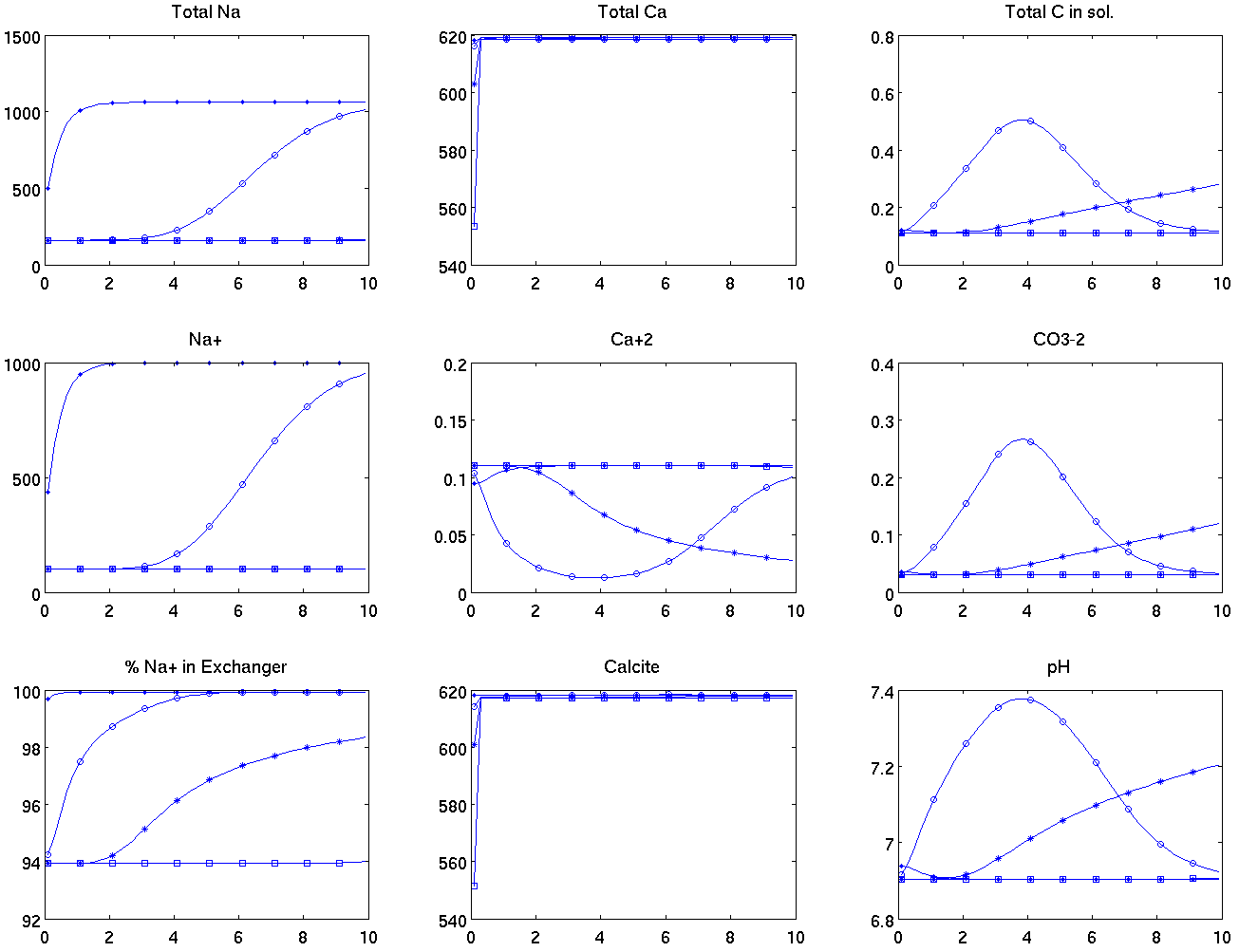#Geochemistry

Solutes, by changing the fluid and rock properties, modify the flow. Water-based EOR methods consist of adding active chemical or biological substances that modify the physical properties of the fluids or/and the porous media at the interface between oil and water. The active EOR chemicals may either increase the sweep efficiency or mobilize the oil. The EOR substances are sensitive to the presence of other chemical components in the fluid, in particular to the pH and the ionic composition of the solution.

Here, we simulate a standard system from geochemistry. We consider a carbon system with three
components (,), calcite dissolution and a ion exchange reaction (,). We have 12 components involved in the following reactionsWe assume instantaneous chemical equilibrium and couple the chemical equation with the flow equations. The governing equations are then the chemical reaction together with the conservation equations for each chemical component (,,) and an inert component which is introduced to enforce electrical balance. In the conservation equations, the complete chemical composition has to be computed to obtain the flux, which correspond to the fluid part of a given element, as the solid part remains in place and does not contribute to the flux.

The chemical equilibrium equations are solved using a method from geometric programming which has been proven to be very efficient in this setting. The fully-coupled system is solved for all the unknown concentrations and pressure. To improve convergence, the chemical equilibrium equations are solved before each Newton step. Such solver requires many assembling of Jacobian matrices. Automatic-differentiation utilities become then very handy as the computation of the Jacobian matrices is done in the background, without explicit implementation.

We consider only one phase flow but the MRST framework enables for easy extension to multi-phase. Here, we present results in 1D but the implementation handles unstructured 3D grid, as the rest of MRST.Siumulation where the concentrations are 10% of those of the residual brine.

The composition of the injected fluid is equal to the resident brine diluted by a factor of ten. This leads to a fluid that is undersatuated with respect to calcite, and as a consequence we see dissolution of calcite at the left of the domain.  At the same time, the composition of the exchanger changes, asis released andis absorbed to equilibrate with the new concentration levels.  We observe the new equilibrium level to be about 94 percent content ofin the exchanger - this level is quickly reached at the influx cell, but only gradually achieved farther downstream, as the brine concentrations there do not quickly adjust to the levels of the injected water.  This inertia arises from interplay between the limited injection rate, the continuous release offrom calcite, and the exchange ofwithby the exchanger.  Simply put, the exchanger needs to absorb significant amounts ofto equilibrate to the new concentrations.  These ions will primarily come from the dissolution of calcite, whose rate of dissolution is here governed by the magnitude of the water flux.  We also observe that the dissolution of calcite is accompanied by a ``wave'' of elevated pH values.  The pH initially rises, then drops again as the system approaches a new steady state upstream.  The new pH values also lead to a different distribution of dissolved carbon, where theconcentration momentarily surpasses that of(is still negligible).

Published September 16, 2014